# Counting the number of cells containing text in Excel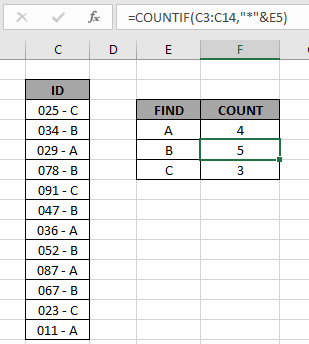In this article, we will learn Counting the number of cells containing text in Excel.

What is COUNTIFS with criteria ?

In simple words, while working with table values, sometimes we need to count the values which ends with a specific text or pattern. Example if we need to find the count of a certain ID which ends with a certain pattern. Criteria inside the formula executed using the operators. Let's see the formula and example to illustrate the formula

How to solve the problem ?

For this article we will be required to use the COUNTIF function. Now we will make a formula out of the function. Here we are given some values in a range and specific text values as criteria. We need to count the values where the formula includes all the values which ends with the given text or pattern

Generic formula:

 = COUNTIF ( range, "*pattern" )

range : values given in as range

* : wildcards which find any number of characters within a given cell.

pattern : given text or pattern to look for. Use pattern directly in the formula or else if you are using cell reference for the pattern use the other formula shown below.

Generic formula:

 = COUNTIF ( range, "*" & pattern )

& : operator to concatenate the 2 values

Example:

All of these might be confusing to understand. So, let's test this formula via running it on the example shown below. Here we have the ID records and we need to find the IDs which ends with A category.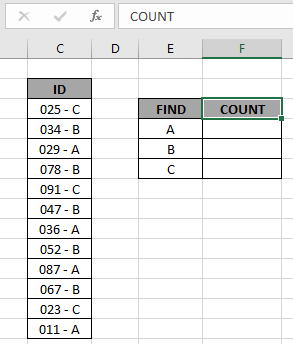Here to find the A category IDs, will be using the * (asterisk) wildcard in the formula. * (asterisk) wildcard finds any number of characters within lookup value.

Use the formula:

 = COUNTIF ( C3:C14, "*A" )

OR

 = COUNTIF ( C3:C14, "*" & E4 )

Explanation:

• COUNTIF function count the cells given criteria
• Criteria is given in using * (asterisk) wildcard to look for value which has any number of characters.
• & operator concatenate the two values where it lays
• "*" & E4 : criteria, formula checks each cell and returns only the cells which satisfies the criteria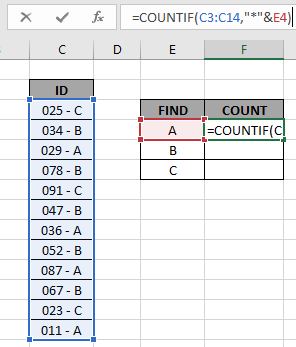Here the range is given as array reference and pattern is given as cell reference. Press Enter to get the count.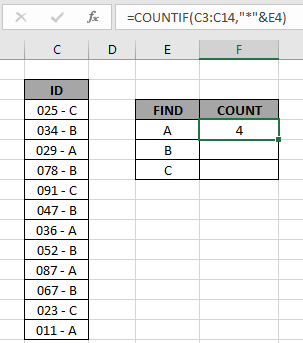As you can see the total values which ends with value A comes out to be 4.

Now copy the formula in other cells using the drag down option or using the shortcut key Ctrl + D as shown below.As you can see all the different category count of IDs is there.

You can check which values end with a specific pattern in range using the excel filter option. Apply the filter to the ID header and click the arrow button which appears. Follow the steps as shown below.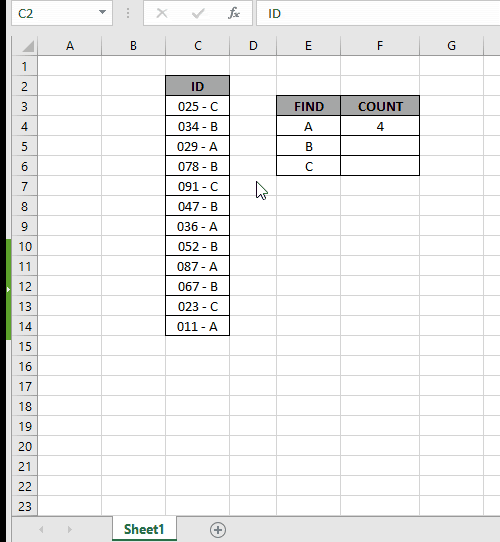Steps:

1. Select the ID header cell. Apply filter using shortcut Ctrl + Shift + L
2. Click the arrow which appeared as a filter option.
3. Deselect (Select All) option.
4. Now search in search box using the * (asterisk) wildcard.
5. Type *A in the search box and select the required values or select the (Select All search result) option as shown in the above gif.

As you can see in the above gif all the 4 values which match the given pattern. This also means the formula works fine to get the count of these values

Here are some observational notes shown below.

Notes:

1. The formula only works with numbers and text both
2. Operators like equals to ( = ), less than equal to ( <= ), greater than ( > ) or not equals to ( <> ) can be performed within function applied with numbers only.

Hope this article about Counting the number of cells containing text in Excel is explanatory. Find more articles on calculating values and related Excel formulas here. If you liked our blogs, share it with your friends on Facebook. And also you can follow us on Twitter and Facebook. We would love to hear from you, do let us know how we can improve, complement or innovate our work and make it better for you. Write to us at info@exceltip.com.

Related Articles :

How to use the SUMPRODUCT function in Excel: Returns the SUM after multiplication of values in multiple arrays in excel.

COUNTIFS with Dynamic Criteria Range : Count cells dependent on other cell values in Excel.

COUNTIFS Two Criteria Match : Count cells matching two different criteria on list in excel.

COUNTIFS With OR For Multiple Criteria : Count cells having multiple criteria match using the OR function.

The COUNTIFS Function in Excel : Count cells dependent on other cell values.

How to Use Countif in VBA in Microsoft Excel : Count cells using Visual Basic for Applications code.

How to use wildcards in excel : Count cells matching phrases using the wildcards in excel.

Popular Articles :

How to use the IF Function in Excel : The IF statement in Excel checks the condition and returns a specific value if the condition is TRUE or returns another specific value if FALSE.

How to use the VLOOKUP Function in Excel : This is one of the most used and popular functions of excel that is used to lookup value from different ranges and sheets.

How to use the SUMIF Function in Excel : This is another dashboard essential function. This helps you sum up values on specific conditions.

How to use the COUNTIF Function in Excel : Count values with conditions using this amazing function. You don't need to filter your data to count specific values. Countif function is essential to prepare your dashboard.

1.